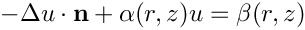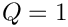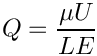oomph::MeshAsGeomObject Class Reference

///////////////////////////////////////////////////////////////////// ///////////////////////////////////////////////////////////////////// More...

#include <mesh_as_geometric_object.h>Inheritance diagram for oomph::MeshAsGeomObject:

## Public Member Functions

SamplePointContainersample_point_container_pt () const
Pointer to the sample point container. More...

FiniteElementfinite_element_pt (const unsigned &e)
Return pointer to e-th finite element. More...

unsigned sample_point_container_version () const
Which sample point container is used in locate zeta? (uses enum Sample_Point_Container_Type) More...

unsigned nelement ()
Number of elements in the underlying mesh. More...

MeshAsGeomObject (Mesh *const &mesh_pt)
Constructor. More...

MeshAsGeomObject (SamplePointContainerParameters *sample_point_container_parameters_pt)
Constructor. More...

MeshAsGeomObject ()
Empty Constructor. More...

~MeshAsGeomObject ()
Destructor. More...

MeshAsGeomObject (const MeshAsGeomObject &)=delete
Broken copy constructor. More...

void operator= (const MeshAsGeomObject &)=delete
Broken assignment operator. More...

unsigned ngeom_data () const
How many items of Data does the shape of the object depend on? More...

Datageom_data_pt (const unsigned &j)
Return pointer to the j-th Data item that the object's shape depends on. More...

void locate_zeta (const Vector< double > &zeta, GeomObject *&sub_geom_object_pt, Vector< double > &s, const bool &use_coordinate_as_initial_guess=false)
Find the sub geometric object and local coordinate therein that corresponds to the intrinsic coordinate zeta. If sub_geom_object_pt=0 on return from this function, none of the constituent sub-objects contain the required coordinate. Following from the general interface to this function in GeomObjects, setting the optional bool argument to true means that each time the sub-object's locate_zeta function is called, the coordinate argument "s" is used as the initial guess. However, this doesn't make sense here and the argument is ignored (though a warning is issued when the code is compiled in PARANOID setting) More...

void position (const Vector< double > &zeta, Vector< double > &r) const
Return the position as a function of the intrinsic coordinate zeta. This provides an (expensive!) default implementation in which we loop over all the constituent sub-objects and check if they contain zeta and then evaluate their position() function. More...

void position (const unsigned &t, const Vector< double > &zeta, Vector< double > &r) const
Parametrised position on object: r(zeta). Evaluated at previous timestep. t=0: current time; t>0: previous timestep. This provides an (expensive!) default implementation in which we loop over all the constituent sub-objects and check if they contain zeta and then evaluate their position() function. More...

void dposition (const Vector< double > &xi, DenseMatrix< double > &drdxi) const
Return the derivative of the position. More...Public Member Functions inherited from oomph::GeomObject
GeomObject ()
Default constructor. More...

GeomObject (const unsigned &ndim)
Constructor: Pass dimension of geometric object (# of Eulerian coords = # of Lagrangian coords; no time history available/needed) More...

GeomObject (const unsigned &nlagrangian, const unsigned &ndim)
Constructor: pass # of Eulerian and Lagrangian coordinates. No time history available/needed. More...

GeomObject (const unsigned &nlagrangian, const unsigned &ndim, TimeStepper *time_stepper_pt)
Constructor: pass # of Eulerian and Lagrangian coordinates and pointer to time-stepper which is used to handle the position at previous timesteps and allows the evaluation of veloc/acceleration etc. in cases where the GeomData varies with time. More...

GeomObject (const GeomObject &dummy)=delete
Broken copy constructor. More...

void operator= (const GeomObject &)=delete
Broken assignment operator. More...

virtual ~GeomObject ()
(Empty) destructor More...

unsigned nlagrangian () const
Access function to # of Lagrangian coordinates. More...

unsigned ndim () const
Access function to # of Eulerian coordinates. More...

void set_nlagrangian_and_ndim (const unsigned &n_lagrangian, const unsigned &n_dim)
Set # of Lagrangian and Eulerian coordinates. More...

TimeStepper *& time_stepper_pt ()
Access function for pointer to time stepper: Null if object is not time-dependent. More...

TimeSteppertime_stepper_pt () const
Access function for pointer to time stepper: Null if object is not time-dependent. Const version. More...

virtual void position (const double &t, const Vector< double > &zeta, Vector< double > &r) const
Parametrised position on object: r(zeta). Evaluated at the continuous time value, t. More...

virtual void dposition_dt (const Vector< double > &zeta, const unsigned &j, Vector< double > &drdt)
j-th time-derivative on object at current time:. More...

virtual void d2position (const Vector< double > &zeta, RankThreeTensor< double > &ddrdzeta) const
2nd derivative of position Vector w.r.t. to coordinates:= ddrdzeta(alpha,beta,i). Evaluated at current time. More...

virtual void d2position (const Vector< double > &zeta, Vector< double > &r, DenseMatrix< double > &drdzeta, RankThreeTensor< double > &ddrdzeta) const
Posn Vector and its 1st & 2nd derivatives w.r.t. to coordinates:= drdzeta(alpha,i).= ddrdzeta(alpha,beta,i). Evaluated at current time. More...

virtual void interpolated_zeta (const Vector< double > &s, Vector< double > &zeta) const
A geometric object may be composed of many sub-objects each with their own local coordinate. This function returns the "global" intrinsic coordinate zeta (within the compound object), at a given local coordinate s (i.e. the intrinsic coordinate of the sub-GeomObject. In simple (non-compound) GeomObjects, the local intrinsic coordinate is the global intrinsic coordinate and so the function merely returns s. To make it less likely that the default implementation is called in error (because it is not overloaded in a derived GeomObject where the default is not appropriate, we do at least check that s and zeta have the same size if called in PARANOID mode. More...

## Private Member Functions

void build_it (SamplePointContainerParameters *sample_point_container_parameters_pt)
Helper function to actually build the thing. More...

## Private Attributes

Vector< Data * > Geom_data_pt
Vector of pointers to Data items that affects the object's shape. More...

Vector< FiniteElement * > Sub_geom_object_pt
Internal storage for the elements that constitute the object. More...

SamplePointContainerSample_point_container_pt
Pointer to the sample point container. More...

OomphCommunicatorCommunicator_pt
Communicator. More...

MeshMesh_pt
Pointer to mesh. More...

unsigned Sample_point_container_version
Which version of the sample point container are we using? More...Protected Attributes inherited from oomph::GeomObject
unsigned NLagrangian
Number of Lagrangian (intrinsic) coordinates. More...

unsigned Ndim
Number of Eulerian coordinates. More...

TimeStepperGeom_object_time_stepper_pt

## Detailed Description

///////////////////////////////////////////////////////////////////// /////////////////////////////////////////////////////////////////////

This class provides a GeomObject representation of a given finite element mesh. The Lagrangian coordinate is taken to be the dimension of the (first) element in the mesh and the Eulerian coordinate is taken to be the dimension of the (first) node in the mesh. If there are no elements or nodes the appropriate dimensions will be set to zero. The constituent elements of the mesh must have their own GeomObject representations, so they must be FiniteElements, and they become sub-objects in this compound GeomObject.

Definition at line 92 of file mesh_as_geometric_object.h.

## ◆ MeshAsGeomObject() [1/4]

 oomph::MeshAsGeomObject::MeshAsGeomObject ( Mesh *const & mesh_pt )
inline

Constructor.

Definition at line 314 of file mesh_as_geometric_object.h.

## ◆ MeshAsGeomObject() [2/4]

 oomph::MeshAsGeomObject::MeshAsGeomObject ( SamplePointContainerParameters * sample_point_container_parameters_pt )
inline

Constructor.

Definition at line 328 of file mesh_as_geometric_object.h.

References build_it().

## ◆ MeshAsGeomObject() [3/4]

 oomph::MeshAsGeomObject::MeshAsGeomObject ( )
inline

Empty Constructor.

Definition at line 336 of file mesh_as_geometric_object.h.

## ◆ ~MeshAsGeomObject()

 oomph::MeshAsGeomObject::~MeshAsGeomObject ( )
inline

Destructor.

Definition at line 339 of file mesh_as_geometric_object.h.

References Sample_point_container_pt.

## ◆ MeshAsGeomObject() [4/4]

 oomph::MeshAsGeomObject::MeshAsGeomObject ( const MeshAsGeomObject & )
delete

Broken copy constructor.

## ◆ build_it()

 void oomph::MeshAsGeomObject::build_it ( SamplePointContainerParameters * sample_point_container_parameters_pt )
inlineprivate

## ◆ dposition()

 void oomph::MeshAsGeomObject::dposition ( const Vector< double > & xi, DenseMatrix< double > & drdxi ) const
inlinevirtual

Return the derivative of the position.

Reimplemented from oomph::GeomObject.

Definition at line 443 of file mesh_as_geometric_object.h.

## ◆ finite_element_pt()

 FiniteElement * oomph::MeshAsGeomObject::finite_element_pt ( const unsigned & e )
inline

Return pointer to e-th finite element.

Definition at line 294 of file mesh_as_geometric_object.h.

References e, and Sub_geom_object_pt.

## ◆ geom_data_pt()

 Data * oomph::MeshAsGeomObject::geom_data_pt ( const unsigned & j )
inlinevirtual

Return pointer to the j-th Data item that the object's shape depends on.

Reimplemented from oomph::GeomObject.

Definition at line 358 of file mesh_as_geometric_object.h.

References Geom_data_pt.

Referenced by build_it().

## ◆ locate_zeta()

 void oomph::MeshAsGeomObject::locate_zeta ( const Vector< double > & zeta, GeomObject *& sub_geom_object_pt, Vector< double > & s, const bool & use_coordinate_as_initial_guess = false )
inlinevirtual

Find the sub geometric object and local coordinate therein that corresponds to the intrinsic coordinate zeta. If sub_geom_object_pt=0 on return from this function, none of the constituent sub-objects contain the required coordinate. Following from the general interface to this function in GeomObjects, setting the optional bool argument to true means that each time the sub-object's locate_zeta function is called, the coordinate argument "s" is used as the initial guess. However, this doesn't make sense here and the argument is ignored (though a warning is issued when the code is compiled in PARANOID setting)

Reimplemented from oomph::GeomObject.

Definition at line 373 of file mesh_as_geometric_object.h.

References SamplePointContainer::locate_zeta(), s, and Sample_point_container_pt.

## ◆ nelement()

 unsigned oomph::MeshAsGeomObject::nelement ( )
inline

Number of elements in the underlying mesh.

Definition at line 308 of file mesh_as_geometric_object.h.

References Sub_geom_object_pt.

## ◆ ngeom_data()

 unsigned oomph::MeshAsGeomObject::ngeom_data ( ) const
inlinevirtual

How many items of Data does the shape of the object depend on?

Reimplemented from oomph::GeomObject.

Definition at line 351 of file mesh_as_geometric_object.h.

References Geom_data_pt.

Referenced by build_it().

## ◆ operator=()

 void oomph::MeshAsGeomObject::operator= ( const MeshAsGeomObject & )
delete

Broken assignment operator.

## ◆ position() [1/2]

 void oomph::MeshAsGeomObject::position ( const unsigned & t, const Vector< double > & zeta, Vector< double > & r ) const
inlinevirtual

Parametrised position on object: r(zeta). Evaluated at previous timestep. t=0: current time; t>0: previous timestep. This provides an (expensive!) default implementation in which we loop over all the constituent sub-objects and check if they contain zeta and then evaluate their position() function.

Reimplemented from oomph::GeomObject.

Definition at line 409 of file mesh_as_geometric_object.h.

## ◆ position() [2/2]

 void oomph::MeshAsGeomObject::position ( const Vector< double > & zeta, Vector< double > & r ) const
inlinevirtual

Return the position as a function of the intrinsic coordinate zeta. This provides an (expensive!) default implementation in which we loop over all the constituent sub-objects and check if they contain zeta and then evaluate their position() function.

Implements oomph::GeomObject.

Definition at line 397 of file mesh_as_geometric_object.h.

References position(), and t.

## ◆ sample_point_container_pt()

 SamplePointContainer * oomph::MeshAsGeomObject::sample_point_container_pt ( ) const
inline

Pointer to the sample point container.

Definition at line 288 of file mesh_as_geometric_object.h.

References Sample_point_container_pt.

## ◆ sample_point_container_version()

 unsigned oomph::MeshAsGeomObject::sample_point_container_version ( ) const
inline

Which sample point container is used in locate zeta? (uses enum Sample_Point_Container_Type)

Definition at line 302 of file mesh_as_geometric_object.h.

References Sample_point_container_version.

## ◆ Communicator_pt

 OomphCommunicator* oomph::MeshAsGeomObject::Communicator_pt
private

Communicator.

Definition at line 275 of file mesh_as_geometric_object.h.

Referenced by build_it().

## ◆ Geom_data_pt

 Vector oomph::MeshAsGeomObject::Geom_data_pt
private

Vector of pointers to Data items that affects the object's shape.

Definition at line 264 of file mesh_as_geometric_object.h.

Referenced by build_it(), geom_data_pt(), and ngeom_data().

## ◆ Mesh_pt

 Mesh* oomph::MeshAsGeomObject::Mesh_pt
private

Pointer to mesh.

Definition at line 280 of file mesh_as_geometric_object.h.

Referenced by build_it(), and position().

## ◆ Sample_point_container_pt

 SamplePointContainer* oomph::MeshAsGeomObject::Sample_point_container_pt
private

Pointer to the sample point container.

Definition at line 270 of file mesh_as_geometric_object.h.

Referenced by build_it(), locate_zeta(), sample_point_container_pt(), and ~MeshAsGeomObject().

## ◆ Sample_point_container_version

 unsigned oomph::MeshAsGeomObject::Sample_point_container_version
private

Which version of the sample point container are we using?

Definition at line 284 of file mesh_as_geometric_object.h.

Referenced by build_it(), and sample_point_container_version().

## ◆ Sub_geom_object_pt

 Vector oomph::MeshAsGeomObject::Sub_geom_object_pt
private

Internal storage for the elements that constitute the object.

Definition at line 267 of file mesh_as_geometric_object.h.

Referenced by build_it(), finite_element_pt(), and nelement().

The documentation for this class was generated from the following file: